# Cloud Pak for Data Group

View Only

## Anomaly Detection in Streams

9 days ago

This article demonstrates how to use the AnomalyDetector operator, which is capable of detecting anomalous subsequences in a streaming time series.

## Introduction

The AnomalyDetector operator is capable of performing online anomaly detection of a time series. More specifically, the AnomalyDetector operator reports anomalies with the pattern of the incoming time series. This type of operator has many different uses and can be utilized in a number of different industries. One example of where this operator may be useful is in the medical industry. By using this operator in conjunction with monitor patients, medical staff can be alerted immediately to changes in patient vital signs.

A time series is a sequence of data points, typically consisting of successive measurements made over a time interval. Examples of time series are ocean tides, counts of sunspots, and the daily closing value of the Dow Jones Industrial Average.Time series - Wikipedia, the free encyclopedia https://en.wikipedia.org/wiki/Time_series

The following image was developed using actual output from the AnomalyDetector operator. As the time series was ingested by the operator, the anomaly detection algorithm analyzed the patterns to determine if there were any anomalies. The orange area was reported by the AnomalyDetector operator as being anomalous.## How it works

The AnomalyDetector operator maintains a recent history of the input time series, which is referred to as the reference pattern. Whenever the AnomalyDetector ingests a tuple, that tuple is added to a buffer called the current pattern (the current pattern is essentially the most recent set of data points received). When this occurs, the operator compares the current pattern with the reference pattern. This comparison operation calculates a score that indicates how similar or dissimilar the current pattern is compared with the reference pattern. The higher the score, the more dissimilar the patterns are. The following example will demonstrate in more detail how the underlying anomaly detection algorithm works.

## Example

In this example, I will provide a high-level demonstration of the algorithm that is used by the AnomalyDetection operator. Rather than discuss every possible parameter, I will focus only on those parameters that are necessary to understand the algorithm. For this example, the following parameter values will be used:referenceLength
```: 10
```
patternLength
```: 3
```
patternCount
`: 5 (default)`
The referenceLength parameter specifies the size of the reference pattern. The patternLength parameter specifies the size of the current pattern. The patternCount parameter specifies how many times the current pattern will be compared against sub-sequences of the reference pattern.

For this example, I will use the following time series. The red square represents the reference pattern, which has a length of 10 (as defined by the referenceLength parameter). The blue square represents the current pattern, which has a length of 3 (as defined by the patternLength parameter).
Note: The boxes represented in the following images include both the start and end data points. For example, the blue box in the image below includes points 8, 9 and 10 (in integral notation, this would be written as [8,10]).The first step is to add a new point to this time series. When the new point is added, the current pattern will be updated to include the new point. (The reference pattern does not get updated until the end, once all of the comparison operations are performed.)Once the new point has been added, the operator will begin comparing the current pattern with sub-sequences of the reference pattern. The sub-sequences will have a length of 3, which are the same size as the current pattern length (defined by the patternLength parameter). The following image demonstrates what the first sub-sequence look like:The above image shows that the first sub-sequence spans 3 points (from 1 to 3, inclusive). The anomaly detection algorithm will compare the sub-sequence reference pattern with the current pattern and calculate a score. Once this has completed, the sub-sequence reference pattern will shift one step to the right and another comparison will be done (the number of steps that the sub-sequence shifts can be set using the stepSize parameter). There will be a total of 5 sub-sequences comparisons performed. The number of comparisons performed is specified by the patternCount parameter. The following images demonstrate the remaining sub-sequence comparisons.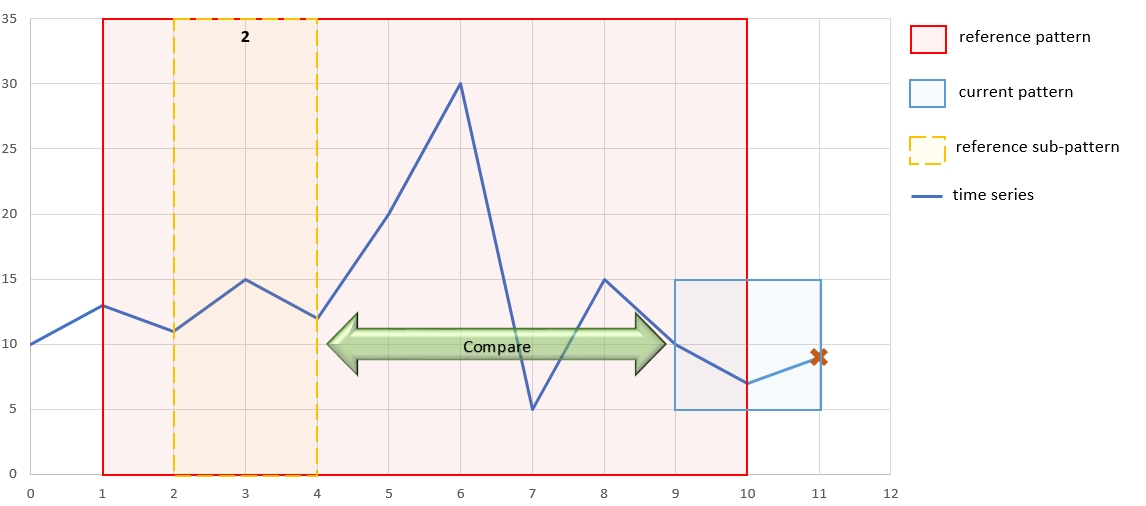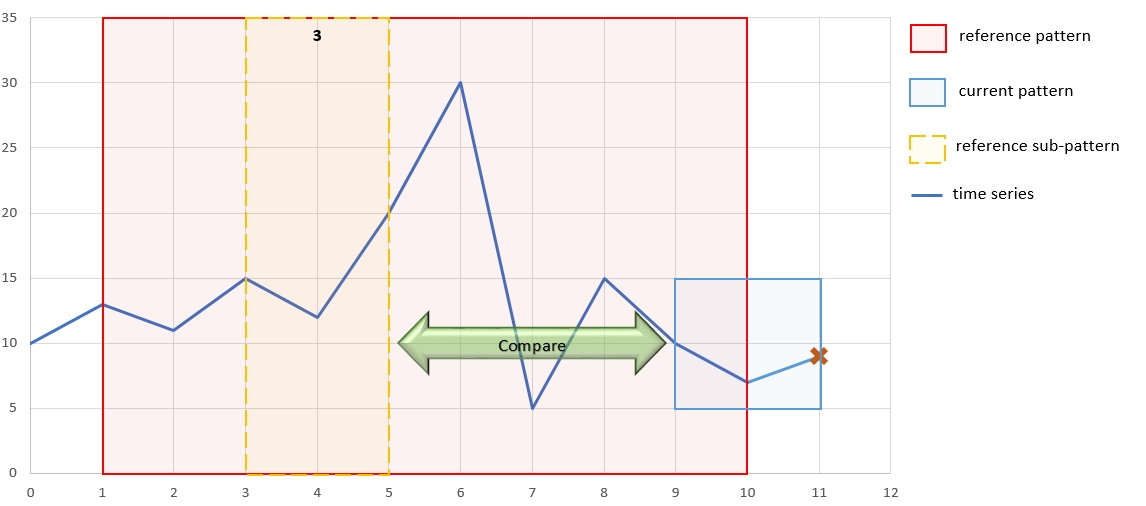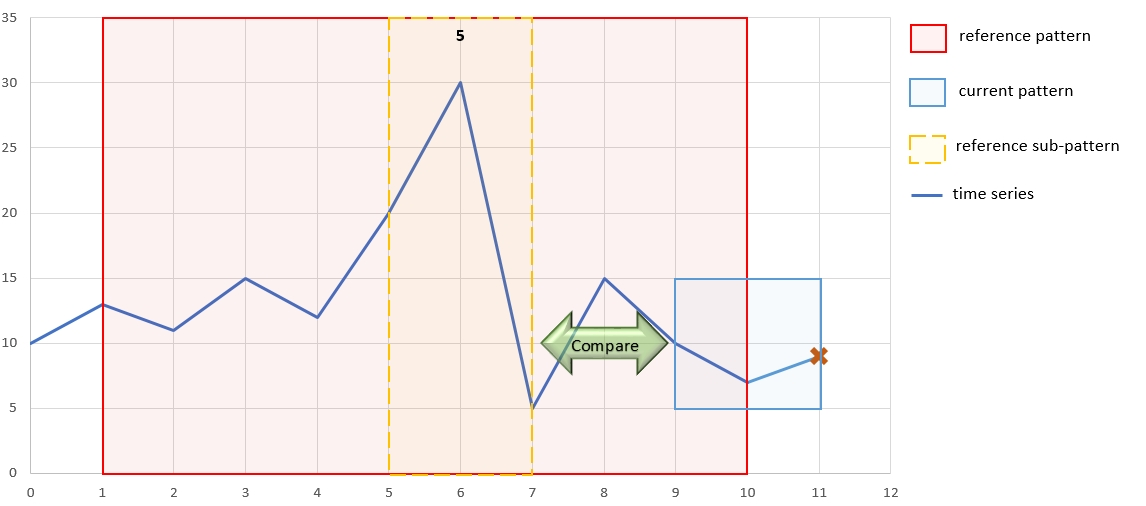Once all of the compare operations have completed, an aggregated score is calculated. This aggregated score is then compared against the value specified by the confidence parameter. If the calculated score is greater than the confidence parameter, the current pattern is considered to be anomalous and the AnomalyDetector operator will submit a tuple containing information about the anomalous pattern. The last step is to update the reference pattern to include the new time series point. Once this is done, the process will repeat.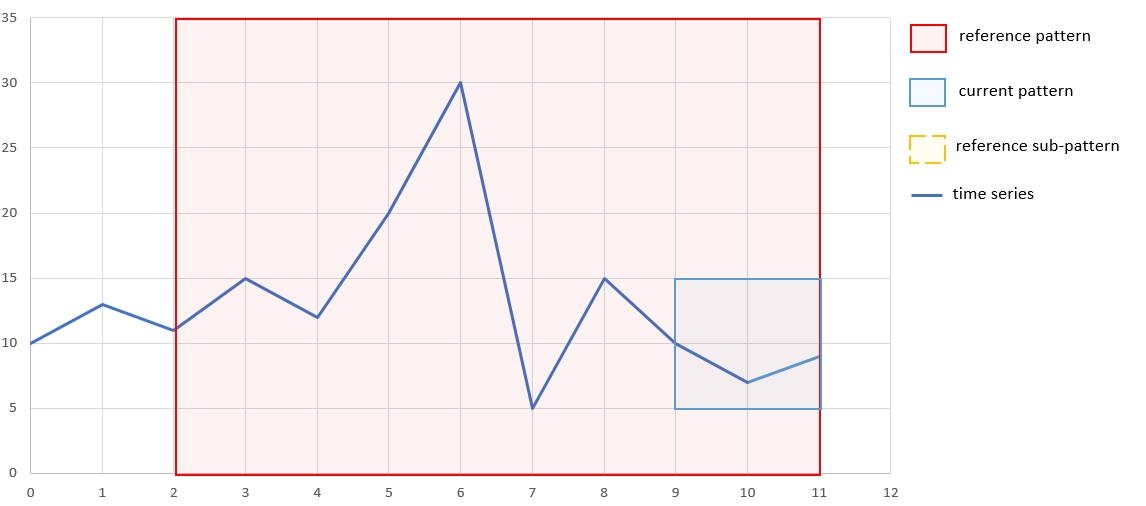## Operator Details

In the previous section, I discussed the underlying algorithm that drives the AnomalyDetector operator. In this section I have provided information about various important aspects of the AnomalyDetector operator. The complete set of documentation for the AnomalyDetector operator can be found on the AnomalyDetector Knowledge Center page.

### Parameters

The AnomalyDetector operator comes with a number of parameters. Details for each of the available parameters can be found on the AnomalyDetector Knowledge Center page. However, there are some important parameters that I want to highlight here.

patternLength - Specifies the length of the 'current pattern' referenceLength - The number of tuples to store as part of the 'reference pattern'
patternCount - The number of subsequence patterns that the current pattern will be compared against stepSize - Specifies how many steps the sliding window will shift (default value is 1)
confidence - Limits the output to only those sequences that have a score equal to or greater than the specified value

### Inputs

The AnomalyDetector operator analyzes a single, continuous time series. The inputTimeseries parameter must be set to an attribute on the input port with a type of float64.

### Outputs

There are four output functions that can be used to return the information about detected anomalies. These output functions include:

getSubsequence() - Returns a list<float64> that contains the anomalous pattern.
getScore() - Returns the calculated score of the anomalous pattern.
getStartTime() - Returns the start time of the anomalous pattern (can only be used if the inputTimestamp parameter is specified) getEndTime() - Returns the end time of the anomalous pattern (can only be used if the inputTimestamp parameter is specified)

## Sample on GitHub

You will find a working sample on GitHub that contains the AnomalyDetector operator: https://github.com/IBMStreams/samples/tree/master/timeseries/AnomalyDetectorSample In this sample, the incoming time series represents the number of packets per second that a NIC received, sampled every second over a 3 minute (180 second) period. Here is an example of what the incoming data looks like:As can be seen from the above, there are 2 obvious anomalies around 60 seconds and 130 seconds. After streaming the data through the AnomalyDetector operator, the following scores (confidence values) were calculated.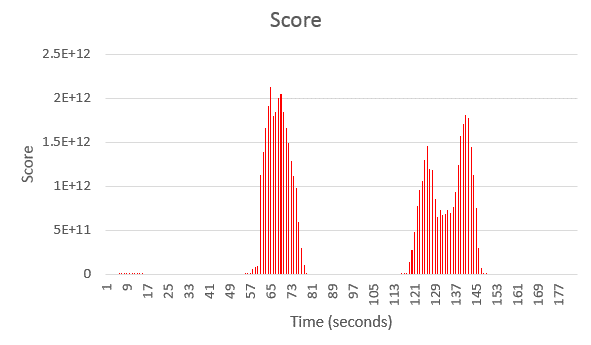From the above chart, we can see that around the same time that the packet count spiked, the score returned by the AnomalyDetector jumps dramatically.

## Conclusion

The AnomalyDetector operator is easy to implement and yet powerful in it's capabilities. The operator is available in the com.ibm.streams.timeseries toolkit packaged with Streams 4.0.0.0 and later.

0 Favorited
24 Views
0 Files
0 Shares